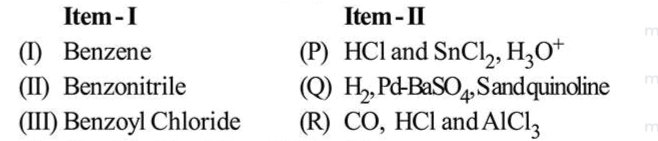# The correct match between Item

Question:

The correct match between Item - I (starting material) and Item - II (reagent) for the preparation of benzaldehyde is :1. (I) - (Q), (II) - (R) and (III) - (P)

2.  (I) - (P), (II) - (Q) and (III) - (R)

3. (I) - (R), (II) - (P) and (III) - (Q)

4. (I) - (R), (II) - (Q) and (III) - (P)

Correct Option:

Solution:

According to Bohr's atomic theory :

(A) Kinetic energy of electron is $\propto \frac{Z^{2}}{n^{2}}$

(B) The product of velocity (v) of electron and principal quantum number (n). 'vn' $\propto Z^{2} .$

(C) Frequency of revolution of electron in an orbit is $\propto \frac{Z^{3}}{n^{3}}$.

(D) Coulombic force of attraction on the electron is $\propto \frac{Z^{3}}{n^{4}}$. Choose the most appropriate answer from the options given below:

(1) (C) only

(2) $(\mathrm{A})$ and $(\mathrm{D})$ only

(3) (A) only

(4) $(\mathrm{A}),(\mathrm{C})$ and $(\mathrm{D})$ only